# Micrometer screw – problems and solutions

1. The measurement of the diameter of the wire with a micrometer is 2.48 mm. The image corresponding to the measurement result is…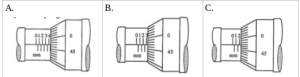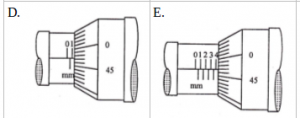Solution :

A. Wire diameter = 4, …..

B. Wire diameter = 3, …..

C. Wire diameter = 2, …..

D. Diameter of wire = 1, …..

E. Wire diameter = 4, …..

[irp]

2. The measurement of the diameter of the wire with a micrometer is 1.95 mm. The image that matches with the measurement results is…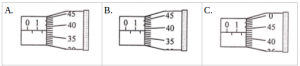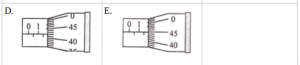Solution :

A.

Main scale = 1.5 mm

Swivel scale = 38 x 0.01 mm = 0.38 mm

0.01 mm is the threshold of micrometer screws accuracy.

Wire diameter = 1.5 mm + 0.38 mm = 1.88 mm.

B.

Main scale = 1.5 mm

Swivel scale = 39 x 0.01 mm = 0.39 mm

0.01 mm is the threshold of micrometer screws accuracy

Wire diameter = 1.5 mm + 0.39 mm = 1.89 mm

C.

Main scale = 1.5 mm

Swivel scale = 43 x 0.01 mm = 0.43 mm

0.01 mm is the threshold of micrometer screws accuracy

Wire diameter = 1.5 mm + 0.43 mm = 1.93 mm

D.

Main scale = 1.5 mm

Swivel scale = 42 x 0.01 mm = 0.42 mm

0.01 mm is the threshold of micrometer screws accuracy

Wire diameter = 1.5 mm + 0.42 mm = 1.92 mm

E.

Main scale = 1.5 mm

Swivel scale = 45 x 0.01 mm = 0.45 mm

0.01 mm is the threshold of micrometer screws accuracy

Wire diameter = 1.5 mm + 0.45 mm = 1.95 mm

[irp]

3. The result of the measurement of the diameter of the metal granule sphere by using screw micrometer is 2.75 mm. The image that matches with the measurement results is…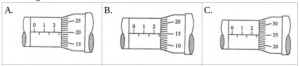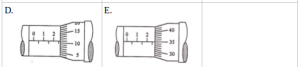Solution :

A.

Main scale = 2.5 mm

Swivel scale = 20 x 0.01 mm = 0.20 mm

Wire diameter = 2.5 mm + 0.20 mm = 2.7 mm

B.

Main scale = 2.5 mm

Swivel scale = 15 x 0.01 mm = 0.15 mm

Wire diameter = 2.5 mm + 0.15 mm = 2.65 mm

C.

Main scale = 2.5 mm

Swivel scale = 25 x 0.01 mm = 0.25 mm

Wire diameter = 2.5 mm + 0.25 mm = 2.75 mm

4. The measurement of the diameter of the steel cylinder with a screw micrometer is 4.47 mm. The correct measurement result shown in the following figure is…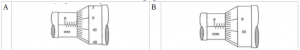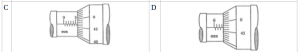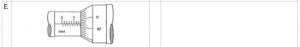Solution :

A.

Main scale = 4.5 mm

Swivel scale = 47 x 0.01 mm = 0.47 mm

0.01 mm is the threshold of micrometer screws accuracy

Wire diameter = 4.5 mm + 0.47 mm = 4.97 mm

B.

Main scale = 4 mm

Swivel scale = 47 x 0.01 mm = 0.47 mm

0.01 mm is the threshold of micrometer screws accuracy

Wire diameter = 4 mm + 0.47 mm = 4.47 mm

C.

Main scale = 5.5 mm

Swivel scale = 47 x 0.01 mm = 0.47 mm

0.01 mm is the threshold of micrometer screws accuracy

Wire diameter = 5.5 mm + 0.47 mm = 5.97 mm

D.

Main scale = 3 mm

Swivel scale = 47 x 0.01 mm = 0.47 mm

0.01 mm is the threshold of micrometer screws accuracy

Wire diameter = 3 mm + 0.47 mm = 3.47 mm

E.

Main scale = 7.5 mm

Swivel scale = 47 x 0.01 mm = 0.47 mm

0.01 mm is the threshold of micrometer screws accuracy

Wire diameter = 7.5 mm + 0.47 mm = 7.97 mm

[irp]